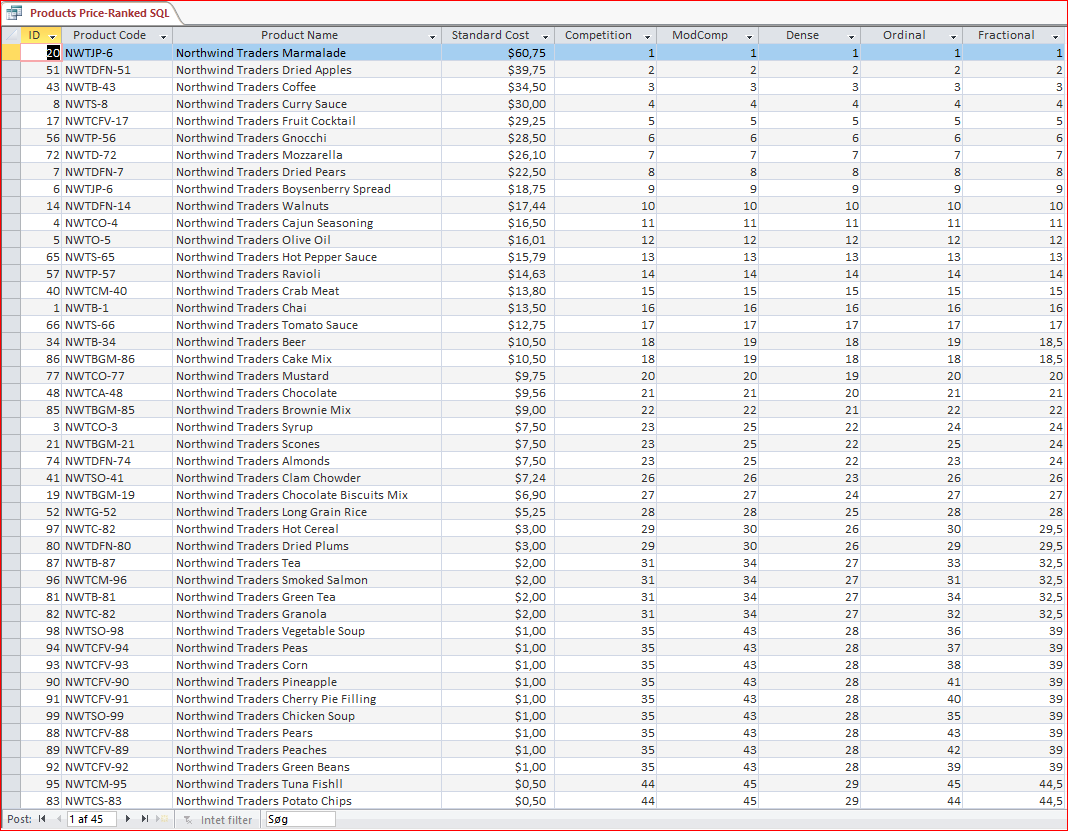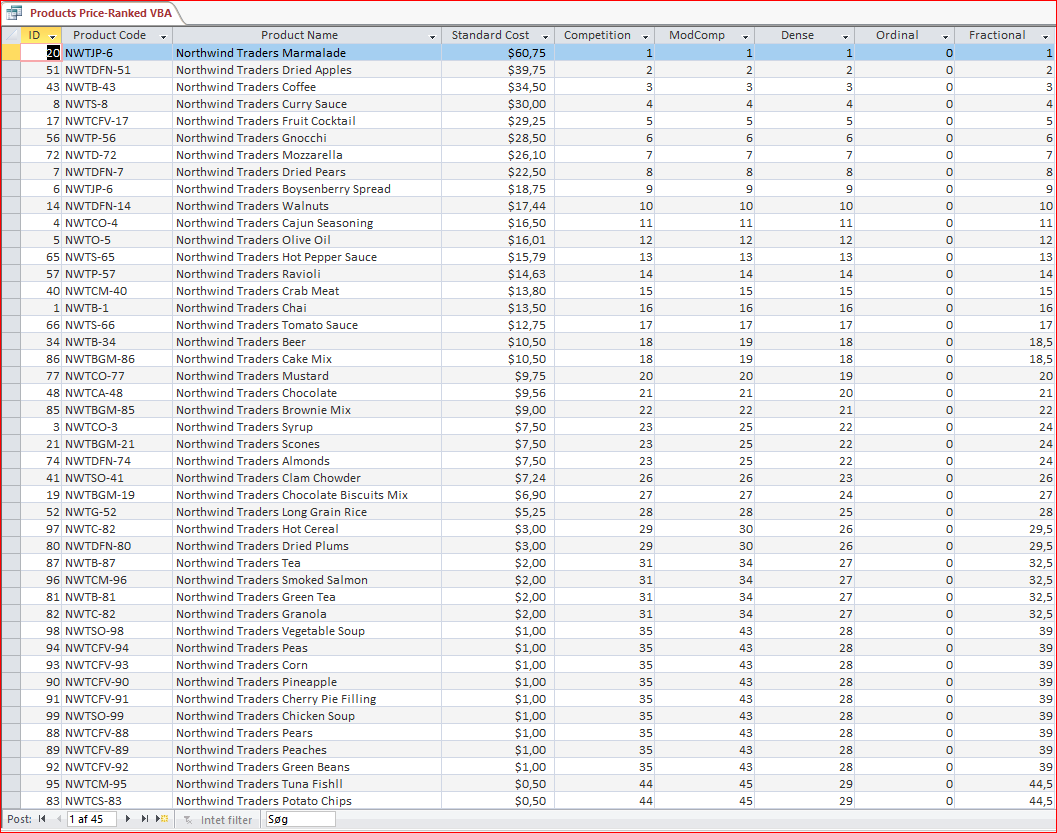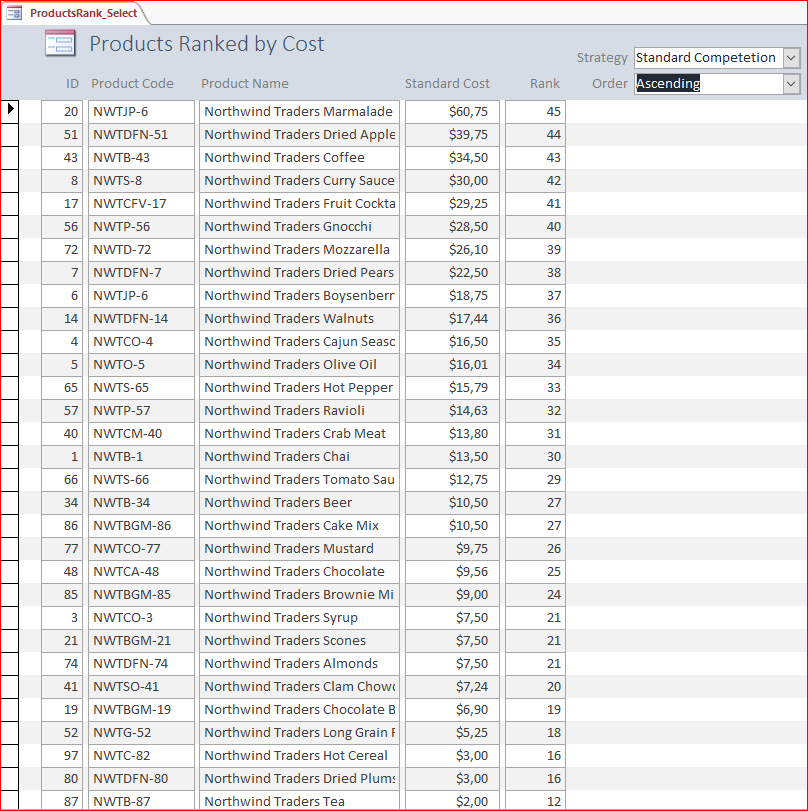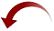# Ranking rows in Microsoft Access

Published on
4,482 Points
282 Views
2 Endorsements
To rank something isn't that difficult. Basically, it is just to sort on the values to rank - points, goals, sales, citizens, whatever - in descending order or (typically for time) ascending order.
But how to rank dupes? Five methods exist, and they can all be implemented in pure SQL or using VBA.

This is the third article on row numbers in Microsoft Access.

The first is about Random Rows in Microsoft Access.

The second is about Sequential Rows in Microsoft Access.

## What to rank?

Many things can be ranked, for example:

• sales results
• prices for a product from different vendors
• fuel consumption for vehicles
• sport results
• eaten hamburgers per quarter

The principle of ranking is not difficult to understand, but one topic complicates matters: Duplicates.
If any two or more of the values are equal, we must have clear guidelines prepared:

• how should the duplicates be ranked?
• how should the duplicates influence the ranking of the succeeding values?

Ranking values, taking this into account, can be done using any of five common methods also called strategies.
Also, the values to rank can be ordered ascending or descending. The default is descending, meaning that the highest values are assigned the highest (numerically lowest) ranks. However, ascending is also common and is what is used for "smallest is best" measures like time to run 100m or fuel consumed per distance unit.

The difference between ascending and descending rank can be illustrated by this small example:

values:
3.4
5.1
2.6
7.3
Ascending
2
3
1
4
Descending
3
2
4
1

## Ranking strategies

A list of these strategies with a detailed explanation for each can be found on WikiPedia: Ranking.
Therefore, here we will only quote the list of strategies and the two matching formulas of Excel in case you are familiar with these:

Name
nick name
excel equivalent formula
Standard competition ranking
"1224" ranking
RANK.EQ
Modified competition ranking
"1334" ranking

Dense ranking
"1233" ranking

Ordinal ranking
"1234" ranking

Fractional ranking
"1 2.5 2.5 4" ranking
RANK.AVG

So, what strategy should you use? For some scenarios, a very strict strategy is either common or has been decided for you. For other scenarios - indeed those of your own - you must choose one. Here it can be of value to be acquainted with the main features for each of these, which are:

strategy
main feature
Standard competition ranking
Identical values are ranked the same
Modified competition ranking
Identical values are ranked the same
Dense ranking
No gaps between the ranks
Ordinal ranking
All record numbers are present, thus all ranks are unique
Fractional ranking
The sum of the ranks is the same as under ordinal ranking

Further:

For the ordinal and the fractional strategies, the sum of the ranks is determined by the count of the values only, not the values.

Finally, in a database, the most difficult strategy to implement is, by far, the ordinal strategy. This is because there is no way to distinguish records with identical field values from each other. As mentioned in the explanation (see link above), a random ranking of such values should not be used, because the ranking should be firm, meaning that repeated calls for the ranks should return identical rankings. Thus, some additional method should be applied.

One method, that will provide predictable results, is to sort on a second field. For a motorrace, for example, this second value could be the driver's grid position, name, or birthdate.

This option has been implemented and will be discussed later.

## Calculate rank with pure SQL

As an example, we can take the Products table of the Northwind example database and rank the products by their cost/price. These contain many duplicates, thus are well suited to illustrate the differences between the ranking strategies.

The SQL of the query will look like this:

```SELECT
Products.*,

1+(Select Count(*)
From Products As T
Where T.[Standard Cost] > Products.[Standard Cost]) AS Competition,

(Select Count(*)
From Products As T
Where T.[Standard Cost] >= Products.[Standard Cost]) AS ModComp,

1+(Select Count(*)
From (Select Distinct S.[Standard Cost] From Products As S) As T
Where T.[Standard Cost] > Products.[Standard Cost]) AS Dense,

(Select Count(*)
From Products As T
Where T.[Standard Cost] >= Products.[Standard Cost])
-(Select Count(*) From Products As S
Where S.[Standard Cost] = Products.[Standard Cost] And S.[Product Code] < Products.[Product Code]) AS Ordinal,

([Competition]+([Competition]
+(Select Count(*)
From Products As T
Where T.[Standard Cost] = Products.[Standard Cost])-1))/2 AS Fractional

FROM
Products
ORDER BY
Products.[Standard Cost] DESC;```

The five strategies have been separated by an empty line, so it should be relatively easy to follow the code, which counts the records and - for each strategy - adds the little twist that makes it unique.

Notice, that for the ordinal strategy - the strategy that needs a second value to sort on - the Product Code has been chosen because then you in plain English can explain why the ranking is like it is. That would not be possible if the ID has been used, as this - even being unique - could appear as more or less random. It could even be hidden from the view as its value - contrary to the Product Code - has no meaning.

The output will be as shown here (click the picture for a better view):You will notice, that the first differences occur for the price of \$10.50, and then these will increase as we move down through the records.

## Calculate rank with VBA

While SQL can be convenient for small recordsets, it may be slow for listing many records because of the subqueries.

Thus, I have created a function, RowRank, using a collection in VBA that will calculate the rank of the records for all five strategies with one table scan only.

The trick is to collect the values in a static collection holding each record's value and an array with the calculated rank for each strategy. Then, for any value and any of the five strategies, the rank can be looked up in a split second.

It takes a little code but, when ready, the advantages are that it is very simple to implement in a form or query, and that it runs truly fast.

It is a single function supported by two enums:

```' Returns, by the value of a field, the rank of one or more records of a table or query.
' Supports all five common ranking strategies (methods).
'
' Source:
'   WikiPedia: https://en.wikipedia.org/wiki/Ranking
'
' Supports ranking of descending as well as ascending values.
' Any ranking will require one table scan only.
' For strategy Ordinal, a a second field with a subvalue must be used.
'
' Typical usage (table Products of Northwind sample database):
'
'   SELECT Products.*, RowRank("[Standard Cost]","[Products]",[Standard Cost]) AS Rank
'   FROM Products
'   ORDER BY Products.[Standard Cost] DESC;
'
' Typical usage for strategy Ordinal with a second field ([Product Code]) holding the subvalues:
'
'   SELECT Products.*, RowRank("[Standard Cost],[Product Code]","[Products]",[Standard Cost],[Product Code],2) AS Ordinal
'   FROM Products
'   ORDER BY Products.[Standard Cost] DESC;
'
' To obtain a rank, the first three parameters must be passed.
' Four parameters is required for strategy Ordinal to be returned properly.
' The remaining parameters are optional.
'
' The ranking will be cached until Order is changed or RowRank is called to clear the cache.
' To clear the cache, call RowRank with no parameters:
'
'   RowRank
'
' Parameters:
'
'   Expression: One field name for other strategies than Ordinal, two field names for this.
'   Domain:     Table or query name.
'   Value:      The values to rank.
'   SubValue:   The subvalues to rank when using strategy Ordinal.
'   Strategy:   Strategy for the ranking.
'   Order:      The order by which to rank the values (and subvalues).
'
' 2019-07-11. Gustav Brock, Cactus Data ApS, CPH.
'
Public Function RowRank( _
Optional ByVal Expression As String, _
Optional ByVal Domain As String, _
Optional ByVal Value As Variant, _
Optional ByVal SubValue As Variant, _
Optional ByVal Strategy As ApRankingStrategy = ApRankingStrategy.apStandardCompetition, _
Optional ByVal Order As ApRankingOrder = ApRankingOrder.apDescending) _
As Double

Const SqlMask1          As String = "Select Top 1 {0} From {1}"
Const SqlMask           As String = "Select {0} From {1} Order By 1 {2}"
Const SqlOrder          As String = ",{0} {1}"
Const OrderAsc          As String = "Asc"
Const OrderDesc         As String = "Desc"
Const FirstStrategy     As Integer = ApRankingStrategy.apDense
Const LastStrategy      As Integer = ApRankingStrategy.apFractional

' Expected error codes to accept.
Const CannotAddKey      As Long = 457
Const CannotFindKey     As Long = 5
' Uncommon character string to assemble Key and SubKey as a compound key.
Const KeySeparator      As String = "¤§¤"

' Array of the collections for the five strategies.
Static Ranks(FirstStrategy To LastStrategy) As Collection
' The last sort order used.
Static LastOrder        As ApRankingOrder

Dim Records             As DAO.Recordset

' Array to hold the rank for each strategy.
Dim Rank(FirstStrategy To LastStrategy)     As Double

Dim Item                As Integer
Dim Sql                 As String
Dim SortCount           As Integer
Dim SortOrder           As String
Dim LastKey             As String
Dim Key                 As String
Dim SubKey              As String
Dim Dupes               As Integer
Dim Delta               As Long
Dim ThisStrategy        As ApRankingStrategy

On Error GoTo Err_RowRank

If Expression = "" Then
' Erase the collections of keys.
For Item = LBound(Ranks) To UBound(Ranks)
Set Ranks(Item) = Nothing
Next
Else
If LastOrder <> Order Or Ranks(FirstStrategy) Is Nothing Then
' Initialize the collections and reset their ranks.
For Item = LBound(Ranks) To UBound(Ranks)
Set Ranks(Item) = New Collection
Rank(Item) = 0
Next

' Build order clause.
Sql = Replace(Replace(SqlMask1, "{0}", Expression), "{1}", Domain)

If Order = ApRankingOrder.apDescending Then
' Descending sorting (default).
SortOrder = OrderDesc
Else
' Ascending sorting.
SortOrder = OrderAsc
End If
LastOrder = Order

' Build SQL.
Sql = Replace(Replace(Replace(SqlMask, "{0}", Expression), "{1}", Domain), "{2}", SortOrder)
' Add a second sort field, if present.
If SortCount >= 2 Then
Sql = Sql & Replace(Replace(SqlOrder, "{0}", 2), "{1}", SortOrder)
End If

' Open ordered recordset.
' Loop the recordset once while creating all the collections of ranks.
While Not Records.EOF
Key = CStr(Nz(Records.Fields(0).Value))
SubKey = ""
' Create the sub key if a second field is present.
If SortCount > 1 Then
SubKey = CStr(Nz(Records.Fields(1).Value))
End If

If LastKey <> Key Then
For ThisStrategy = FirstStrategy To LastStrategy
Select Case ThisStrategy
Case ApRankingStrategy.apDense
Rank(ThisStrategy) = Rank(ThisStrategy) + 1
Case ApRankingStrategy.apStandardCompetition
Rank(ThisStrategy) = Rank(ThisStrategy) + 1 + Dupes
Dupes = 0
Case ApRankingStrategy.apModifiedCompetition
Rank(ThisStrategy) = Rank(ThisStrategy) + 1
Case ApRankingStrategy.apOrdinal
Rank(ThisStrategy) = Rank(ThisStrategy) + 1
' Add entry using both Key and SubKey
Ranks(ThisStrategy).Add Rank(ThisStrategy), Key & KeySeparator & SubKey
Case ApRankingStrategy.apFractional
Rank(ThisStrategy) = Rank(ThisStrategy) + 1 + Delta / 2
Delta = 0
End Select
If ThisStrategy = ApRankingStrategy.apOrdinal Then
' Key with SubKey has been added above for this strategy.
Else
' Add key for all other strategies.
End If
Next
LastKey = Key
Else
' Modify entries and/or counters for those strategies that require this for a repeated key.
For ThisStrategy = FirstStrategy To LastStrategy
Select Case ThisStrategy
Case ApRankingStrategy.apDense
Case ApRankingStrategy.apStandardCompetition
Dupes = Dupes + 1
Case ApRankingStrategy.apModifiedCompetition
Rank(ThisStrategy) = Rank(ThisStrategy) + 1
Ranks(ThisStrategy).Remove Key
Case ApRankingStrategy.apOrdinal
Rank(ThisStrategy) = Rank(ThisStrategy) + 1
' Will fail for a repeated value of SubKey.
Ranks(ThisStrategy).Add Rank(ThisStrategy), Key & KeySeparator & SubKey
Case ApRankingStrategy.apFractional
Rank(ThisStrategy) = Rank(ThisStrategy) + 0.5
Ranks(ThisStrategy).Remove Key
Delta = Delta + 1
End Select
Next
End If
Records.MoveNext
Wend
Records.Close
End If

' Retrieve the rank for the current strategy.
If Strategy = ApRankingStrategy.apOrdinal Then
' Use both Value and SubValue.
Key = CStr(Nz(Value)) & KeySeparator & CStr(Nz(SubValue))
Else
' Use Value only.
Key = CStr(Nz(Value))
End If
' Will fail if key isn't present.
Rank(Strategy) = Ranks(Strategy).Item(Key)
End If

RowRank = Rank(Strategy)

Exit_RowRank:
Exit Function

Err_RowRank:
Select Case Err
' Key is present, thus cannot be added again.
Resume Next
Case CannotFindKey
' Key is not present, thus cannot be removed.
Resume Next
Case Else
' Some other error. Ignore.
Resume Exit_RowRank
End Select

End Function```

These are the enums to complete the picture:

```'   Ranking strategies. Numeric values match those of:
'   https://se.mathworks.com/matlabcentral/fileexchange/70301-ranknum
Public Enum ApRankingStrategy
apDense = 1
apOrdinal = 2
apStandardCompetition = 3
apModifiedCompetition = 4
apFractional = 5
End Enum

'   Ranking orders.
Public Enum ApRankingOrder
apDescending = 0
apAscending = 1
End Enum```

Now, using this function, we can build a query returning exactly the same output as the query above:

```SELECT
Products.*,
RowRank("[Standard Cost],[Product Code]","Products",[Standard Cost],[Product Code],3) AS Competition,
RowRank("[Standard Cost],[Product Code]","Products",[Standard Cost],[Product Code],4) AS ModComp,
RowRank("[Standard Cost],[Product Code]","Products",[Standard Cost],[Product Code],1) AS Dense,
RowRank("[Standard Cost],[Product Code]","Products",[Standard Cost],[Product Code],2) AS Ordinal,
RowRank("[Standard Cost],[Product Code]","Products",[Standard Cost],[Product Code],5) AS Fractional
FROM
Products
ORDER BY
Products.[Standard Cost] DESC;```

You'll notice how much simpler it is.

Also note, that the secondary value - the Product Code - has been included, as we wish to also list the ordinal rank. From the in-line comments (at the top of the code block) you'll see, that the simplest default implementation is:

```SELECT Products.*, RowRank("[Standard Cost]","[Products]",[Standard Cost]) AS Rank
FROM Products
ORDER BY Products.[Standard Cost] DESC;```

Just for verification, the output of the extended query is:Cached ranking

As the ranks are cached - they are stored in the collection - you may wish to reset the ranking (clear the cache). This can be done in two ways:

• call RowRank with another sort order, or:
• call RowRank with no arguments at all:

`RowRank`

## Ranking records of a form

For many purposes, you may wish to calculate and display the ranks directly in a form without having to build a special query. You may even wish to select between the five strategies on the fly.

This is easily done using the function.  Just add a textbox with this expression as ControlSource:

`=RowRank("[Standard Cost],[Product Code]","Products",[Standard Cost],[Product Code],[Strategy],[RankOrder])`

Add two combo-boxes (here named Strategy and RankOrder) to select the strategy and sort order, and the result may appear like:Change the strategy or the sort order, and the form will requery the ranks immediately.

#### Cached ranking

Too make sure that the cache is cleared before opening the form, you may include this single line of code in the Load event of the form:

```Private Sub Form_Load()

' Reset all ranks.
RowRank

End Sub```

As a clean-up, it can be included in the UnLoad event as well.

## Sorting on rank in a form

Most often you will have sorted the form on the field to rank. But if you can't do that and wish to sort on the rank itself, it must be calculated in the source query of the form. This means, that the query has a field holding the rank, and that a textbox on the form is bound to this.

However, if you sort on the field to rank, the textbox holding the rank will automatically be sorted. This opens for alternative methods for calculating the rank.

First, as shown above, the RowRank function can be used directly as the ControlSource, for example:

`=RowRank("[Standard Cost]","Products",[Standard Cost],Null,5)`

Second, and mostly for completeness, you can use the domain function DCount, though it often will be too slow:

`=1+DCount("*","[Products]","[Standard Cost]>" & Str([Standard Cost]) & "")`

Here, the purpose of Str is to force a proper format of a decimal value with a dot as the decimal separator.

## Demo

The attached demo application (Access 2016) contains, of course, the code as listed as well as four forms  covering everything discussed above.

Also, the two queries - using SQL and VBA respectively - are included for you to check out:

RowNumbers 1.4.1.zip

Current code is also on GitHub: VBA.RowNumbers

You may also enjoy my previous articles covering all other aspects of row/record numbering:

Random Rows in Microsoft Access

Sequential Rows in Microsoft Access